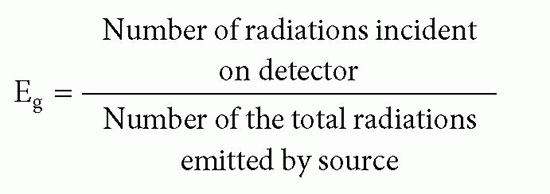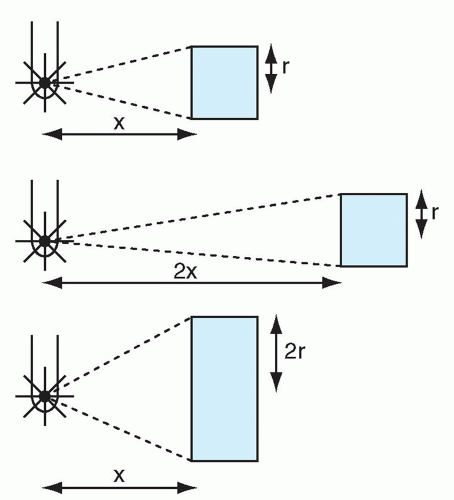# In Vitro Radiation Detection

In Vitro Radiation Detection

In many nuclear medicine procedures, such as the Schilling test, blood volume determinations, protein and fat absorption studies, ferrokinetics, and various radioimmunoassay, it is necessary to determine the amount of radioactivity in a given sample as compared with a standard. In some of these tests (e.g., Schilling test), a radioactive substance is administered to a patient; in others, such as various radioimmunoassay, no radioactive compound is given to the patient. In both cases, however, the choice of the radiation detector and the geometric setup for the measurement of a given radioactive sample are of great importance and are primarily dictated by the overall efficiency (sensitivity) of a particular setup. An increased overall efficiency in these studies allows one to reduce either the radiation dose to a patient or the count time for a given standard deviation error.

This chapter describes the various techniques used for the in vitro detection of the radioactivity of both β-ray and γ-ray emitting radionuclides.

Overall Efficiency E

The overall efficiency E of a given device for the measurement of radioactivity depends on the geometric efficiency of the physical setup Eg and the intrinsic efficiency Ei of the detector. Thus, the overall efficiency can be expressed as

E = Eg × Ei

Intrinsic Efficiency. The intrinsic efficiency Ei (see Chapter 8) is defined asIt primarily depends on the linear attenuation coefficient µ (linear) of the material of which a detector is made and on the thickness of the detector.

In a scintillation counter, when one restricts oneself to the photo peak counts only, the resulting intrinsic efficiency is known as the photo peak efficiency.

Geometric Efficiency. Geometric efficiency Eg can be understood as follows. Let a small sample of radioactive compound be located at a distance from a detector with a cross-sectional radius r as shown in Figure 9.1 (top). Because there is no preferred direction for the emission of radiation from a radionuclide, the radiations (α, β, or γ) from a radioactive sample will be emitted with equal probability in all directions. Therefore, of the total number of nuclear radiations emitted in all directions, only a fraction of these will be incident on the sensitive volume of the detector (Fig. 9.1, top). Geometric efficiency Eg, then, is defined as the ratio of the number of radiations incident on the detector from a radioactive sample
to the total number of radiations emitted by the sample, orThe geometric efficiency Eg, for the device shown in Figure 9.1 (top), depends on two factors: the distance x between the source and the detector, and the radius r of the cross-sectional area of the detector. For distances that are sufficiently larger than the radius of the detector (i.e., x >> r), the geometric efficiency varies as 1/x2 and r2, respectively, with the distance x and the radius r; that is, if one increases the distance between the sample and the detector twofold, the geometric efficiency is reduced fourfold (Fig. 9.1, center). If the radius of the detector is doubled, the geometric efficiency will be increased fourfold (Fig. 9.1, bottom).Figure 9.1. Variations in geometric efficiency of a single radiation detector in three different geometric arrangements (top, center, and bottom). A radioactive source emits radiations in all directions. Only those radiations that travel in the direction enclosed by the dotted lines reach the detector. The geometric efficiency, which is a measure of the number of γ-rays that reach the detector, depends on the distance x between the source and the detector and the radius of the detector as 1/x2 and r2. Thus, the geometric efficiency of center arrangement is one-fourth of top arrangement; the geometric efficiency of bottom arrangement is four times that of top arrangement. In such an arrangement, however, geometric efficiency can never be higher than 50%.

When the sample is very close to the detector (i.e., x << r), the above relationship Eg ∝ (1/x2) does not hold true. The exact relationship between Eg and the distance x, in this case, is of no practical concern to us here except to know that the maximum obtainable geometric efficiency is achieved when x = 0 (i.e., the source is directly against the face of the detector). In this case, Eg approaches 50%, because even in this situation, one-half of the radiations are emitted away from the detector.

To increase Eg to more than 50%, one has to either use more than one detector or somehow surround the sample from all sides by the detector. The second approach is used in well-type NaI(Tl) scintillation detectors (well counters) and liquid scintillation detectors described later and is also used in dose calibrators as is described in Chapter 8.

Well-Type NaI(Tl) Scintillation Detectors (Well Counters)

In terms of its operation and associated electronics, the well-type NaI(Tl) scintillation detector (well counter) is exactly the same as the NaI(Tl) scintillation detector described in Chapter 8, except that in a well counter, a small cylindrical hole is constructed in the NaI(Tl) crystal to allow a radioactive sample to be positioned very close to the center of the crystal (Fig. 9.2). In this type of detector, only a small fraction (<5%) of the radiations emitted by the sample, escapes from the sensitive volume of the crystal. Thus, Eg approaches 95%. The intrinsic efficiency of an NaI(Tl) detector is dependent on the size of the crystal—the larger the crystal, the higher the intrinsic efficiency for a given energy γ-ray. Well counters are commercially available in various sizes. The so-called standard well counter (1.75-inch diameter, 2 inches in height with a hole 0.75 inches in diameter and 1.5 inches deep) enjoys the most popularity in nuclear medicine. For high-energy γ-rays (>500 keV), however, one achieves a far better count rate with a 3 × 3-inch well counter
than with the standard model. Table 9.1 lists the intrinsic efficiencies of standard and 3 × 3-inch well counters for various γ-ray energies. It can be seen from columns 2 and 3 that at higher energies, one gains a factor of 1.5 in intrinsic efficiency by using a 3 × 3-inch rather than a standard well counter. For many procedures, one is interested only in the photo peak counts (i.e., one rejects γ-ray interactions via the Compton scattering). In this case, the advantage of a 3 × 3-inch over a standard well counter for higher energy γ-rays becomes even greater (see columns 4 and 5 in Table 9.1).Figure 9.2. Geometric efficiency of a well-type detector. The NaI crystal is shown in gray color. Only a small fraction of radiations that are emitted in the direction enclosed by two dotted lines miss the detector. In such an arrangement, geometric efficiency is close to 95%.

Table 9.1 Efficiency of a Well Counter

 Intrinsic Efficiency Photo Peak Efficiency Energy (keV) Standard Well 3 × 3 Well Standard Well 3 × 3 Well 80 97a 98a 97a 98a 140 94 98 88 96 280 61 80 49 70 320 51 73 36 59 360 48 68 31 50 410 43 66 24 45 510 38 59 17 36 660 32 51 12 25 880 29 46 8 17 1,110 28 45 7 16 1,170 25 42 6 15 1,270 24 40 5 14 a Attenuation in sample and sample holder will reduce these numbers slightly.

Only gold members can continue reading. Log In or Register to continue

Nov 8, 2018 | Posted by in GENERAL SURGERY | Comments Off on In Vitro Radiation Detection
Premium Wordpress Themes by UFO Themes